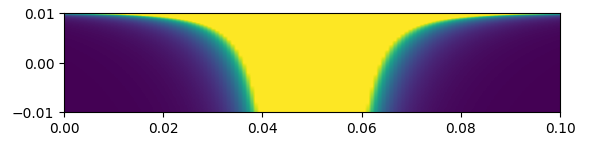# Arbitrary Reparameterization of Model

Is there a clean way to reparameterize a continuous model in numpyro using handlers for arbitrary transformations? i.e. I have some model f(x,y), and some other set of parameters u(x,y), v(x,y), and I want to transform f(x,y) to a new probability density in terms of u and v. I know the mathematical transformation is simply a correction by det|J|:

g(u,v) = f\left (x(u,v),y(u,v) \right) \cdot det \lvert J(u,v) \rvert

Where J(u,v) is the jacobian of the transformation (u,v) \rightarrow (x,y). Obviously, I can do this using log_density and some messy workarounds, e.g. suppose I have u=(x+2y)^{-3} and v=(x-2y)^{-1}, I can do as shown below, but this hardly seems like the cleanest way to do things.

What is the recommended procedure for constructing and applying such transforms in NumPyro?

# Forwards Transformation: x,y - > u,v
def uf(x,y):
return (x+2*y)**3

def vf(x,y):
return(1/(x-2*y))

def Jxy(x,y):
out = jnp.linalg.det(out)
return out

#-----------------

# Backwards Transformation: u,v - > x,y
def xf(u,v):
return 1/2 * (1/u**(1/3) + 1/v)

def yf(u,v):
return 1/4 * (1/u**(1/3) - 1/v)

def Juv(u,v):
out = jnp.linalg.det(out)
return out

#----------------
# Models
def model():
'''Base Model, f(x,y)'''
numpyro.sample('x', dist.Normal(0,1))
numpyro.sample('y', dist.HalfNormal(2))

@jax.jit
def like_uv(u,v):
x, y = xf(u,v), yf(u,v)
detJ = Juv(u,v)
logd_xy = numpyro.infer.util.log_density(model, (), {}, {'x':x,'y':y})
out = logd_xy*detJ
return(out)

def model_uv():
'''Transformed Model, g(u,v)'''
u = numpyro.sample('u', dist.ImproperUniform(dist.constraints.real, (), ()))
v = numpyro.sample('v', dist.ImproperUniform(dist.constraints.real, (), ()))

numpyro.factor('transformed_density', like_uv(u,v) )

@jax.jit
def like_uv2(u,v):
return numpyro.infer.util.log_density(model_uv, (), {}, {'u':u, 'v':v})



Note, I’ve tested the above and confirmed that like_uv and like_uv2’ do produce the same probability density:You can define a Transform for it. Then, to reparameterize, you can use TransformDistribution together with TransformReparam.

I’m having a bit of a hard time following the documentation on this. I start by creating a Transform object, which I understand acts as a wrapper for my various transformation functions:

from numpyro.distributions.transforms import ParameterFreeTransform

class xyuv_transform(ParameterFreeTransform):

def __call__(self, X):
x, y = X, X
u, v = uf(x,y), vf(x,y)
return(jnp.array([u,v]))

def _inverse(self, U):
u, v = U, U
x, f = xf(u,v), yf(u,v)
return(jnp.array([x,y]))

def log_abs_det_jacobian(self, x, y, intermediates=None):
out = jnp.linalg.det(out)
return out


But how do I proceed from here? I know I will at some stage need to call:

with reparam( config = {
'x': TransformReparam(),
'y': TransformReparam()},
)


Along with:

TransformedDistribution([SOME DISTRIBUTION], xyuv_transform)


But the details are escaping me. All of the documented examples are for 1D cases (e.g. 1, 2), while I want to transform two sites at once. Ideally I’d like to do this without altering anything in model_xy(), as though it were a black box. I understand I could do this using handlers.condition to enforce values of x and y onto model, but this still feels like a messy work-around

You’ll need to define domain and codomain properly. Unfortunately, we don’t have mixed domains for (Real, Real_plus) and mixed distributions of (Normal, HalfNormal). I’m not sure what’s a good way to support arbitrary transformations like the one above.

What would be the correct procedure if all distributions in x and y were unconstrained?

You will need to change domain, codomain of your transform to RealVector instead of Vector`. The rest should be the same as in other examples that you linked.